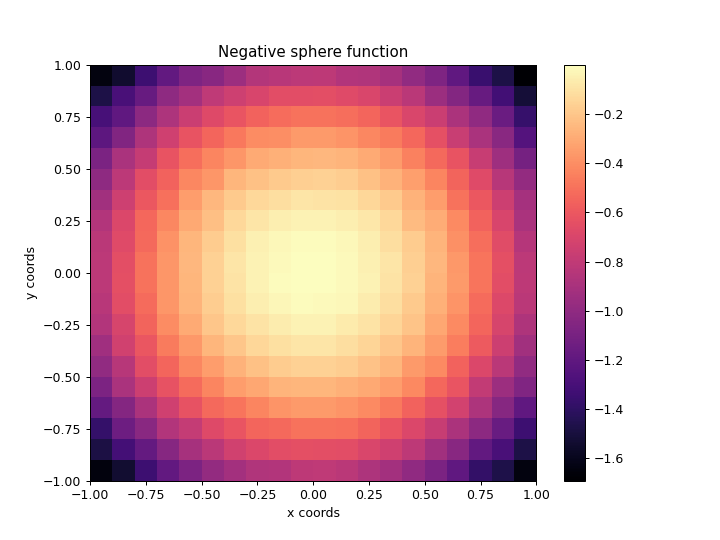# ribs.visualize.grid_archive_heatmap¶

ribs.visualize.grid_archive_heatmap(archive, ax=None, transpose_bcs=False, cmap='magma', square=False, vmin=None, vmax=None, pcm_kwargs=None)[source]

Plots heatmap of a GridArchive with 2D behavior space.

Essentially, we create a grid of cells and shade each cell with a color corresponding to the objective value of that cell’s elite. This method uses pcolormesh() to generate the grid. For further customization, pass extra kwargs to pcolormesh() through the pcm_kwargs parameter. For instance, to create black boundaries of width 0.1, pass in pcm_kwargs={"edgecolor": "black", "linewidth": 0.1}.

Examples

>>> import numpy as np
>>> import matplotlib.pyplot as plt
>>> from ribs.archives import GridArchive
>>> from ribs.visualize import grid_archive_heatmap
>>> # Populate the archive with the negative sphere function.
>>> archive = GridArchive([20, 20], [(-1, 1), (-1, 1)])
>>> archive.initialize(solution_dim=2)
>>> for x in np.linspace(-1, 1, 100):
...     for y in np.linspace(-1, 1, 100):
...                     objective_value=-(x**2 + y**2),
...                     behavior_values=np.array([x,y]))
>>> # Plot a heatmap of the archive.
>>> plt.figure(figsize=(8, 6))
>>> grid_archive_heatmap(archive)
>>> plt.title("Negative sphere function")
>>> plt.xlabel("x coords")
>>> plt.ylabel("y coords")
>>> plt.show()Parameters
• archive (GridArchive) – A 2D GridArchive.

• ax (matplotlib.axes.Axes) – Axes on which to plot the heatmap. If None, the current axis will be used.

• transpose_bcs (bool) – By default, the first BC in the archive will appear along the x-axis, and the second will be along the y-axis. To switch this (i.e. to transpose the axes), set this to True.

• cmap (str, list, matplotlib.colors.Colormap) – Colormap to use when plotting intensity. Either the name of a colormap, a list of RGB or RGBA colors (i.e. an Nx3 or Nx4 array), or a colormap object.

• square (bool) – If True, set the axes aspect ratio to be “equal”.

• vmin (float) – Minimum objective value to use in the plot. If None, the minimum objective value in the archive is used.

• vmax (float) – Maximum objective value to use in the plot. If None, the maximum objective value in the archive is used.

• pcm_kwargs (dict) – Additional kwargs to pass to pcolormesh().

Raises

ValueError – The archive is not 2D.